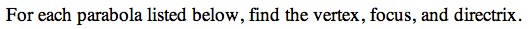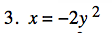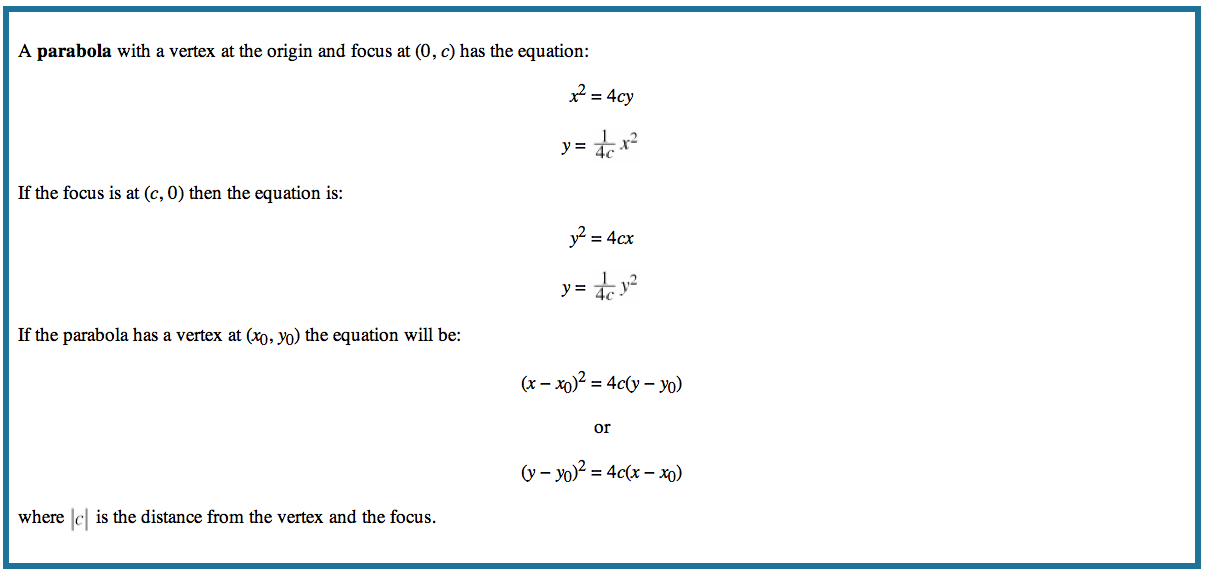13P-3.

### Missing problem for Book PCT => Chapter Ch13 => Lesson 13.1.4 => Problem 13P-3

Created from orphaned homework help problemIf x = 1/(4c)y², what is c based on your given equation?